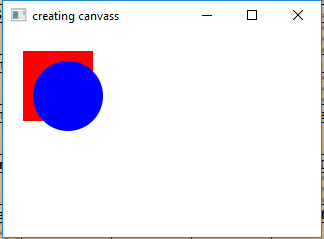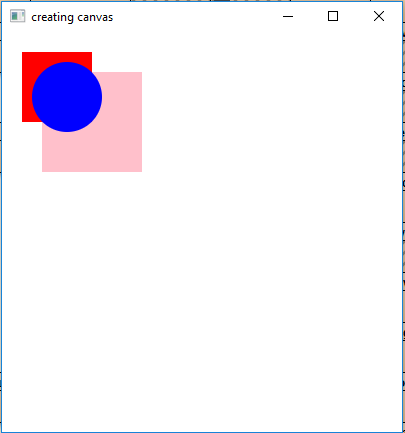# JavaFX | Canvas Class

Canvas class is a part of JavaFX. Canvas class basically creates an image that can be drawn on using a set of graphics commands provided by a GraphicsContext. Canvas has a specified height and width and all the drawing operations are clipped to the bounds of the canvas.

Constructors of the class:

1. Canvas(): Creates a new canvas object.
2. Canvas(double w, double h): Creates a new canvas object with specified width and height.

Commonly Used Methods:

Method Explanation
getGraphicsContext2D() Returns the graphics context associated with the canvas.
getHeight() Returns the height of the canvas.
getWidth() Returns the width of the canvas.
setHeight(double v) Sets the height of the canvas.
setWidth(double d) Sets the width of the canvas.

Below programs illustrate the use of Canvas class:

1. Java Program to create a canvas with specified width and height(as arguments of constructor), add it to the stage and also add a circle and rectangle on it: In this program we will create a Canvas named canvas with specified width and height. We will extract the GraphicsContext using the getGraphicsContext2D() function and draw a rectangle and a oval of different color. Now we will create a Group named group and add the canvas to the group. Now create a scene and add the group to the scene and then attach the scene to the stage and call the show() function to display the results.

 `// Java Program to create a canvas with specified ` `// width and height(as arguments of constructor), ` `// add it to the stage and also add a circle and ` `// rectangle on it ` `import` `javafx.application.Application; ` `import` `javafx.scene.Scene; ` `import` `javafx.scene.control.*; ` `import` `javafx.scene.layout.*; ` `import` `javafx.stage.Stage; ` `import` `javafx.event.ActionEvent; ` `import` `javafx.event.EventHandler; ` `import` `javafx.scene.canvas.*; ` `import` `javafx.scene.paint.Color; ` `import` `javafx.scene.Group; ` ` `  `public` `class` `canvas ``extends` `Application { ` ` `  `    ``// launch the application ` `    ``public` `void` `start(Stage stage) ` `    ``{ ` ` `  `        ``// set title for the stage ` `        ``stage.setTitle(``"creating canvas"``); ` ` `  `        ``// create a canvas ` `        ``Canvas canvas = ``new` `Canvas(``100``.0f, ``100``.0f); ` ` `  `        ``// graphics context ` `        ``GraphicsContext graphics_context =  ` `             ``canvas.getGraphicsContext2D(); ` ` `  `        ``// set fill for rectangle ` `        ``graphics_context.setFill(Color.RED); ` `        ``graphics_context.fillRect(``20``, ``20``, ``70``, ``70``); ` ` `  `        ``// set fill for oval ` `        ``graphics_context.setFill(Color.BLUE); ` `        ``graphics_context.fillOval(``30``, ``30``, ``70``, ``70``); ` ` `  `        ``// create a Group ` `        ``Group group = ``new` `Group(canvas); ` ` `  `        ``// create a scene ` `        ``Scene scene = ``new` `Scene(group, ``200``, ``200``); ` ` `  `        ``// set the scene ` `        ``stage.setScene(scene); ` ` `  `        ``stage.show(); ` `    ``} ` ` `  `    ``// Main Method ` `    ``public` `static` `void` `main(String args[]) ` `    ``{ ` ` `  `        ``// launch the application ` `        ``launch(args); ` `    ``} ` `} `

Output:2. Java Program to create a canvas and use setHeight() and setWidth() function to set canvas size and add it to the stage and also add a circle and rectangle on it: In this program we will create a Canvas named canvas and set the width and height using the setWidth() and setHeight() function. We will extract the GraphicsContext using the getGraphicsContext2D() function and draw two rectangles and a oval of different color. We will create a Group named group and add the canvas to the group. We will create a scene and add the group to the scene and then attach the scene to the stage. Finally, call the show() function to display the results.

 `// Java Program to create a canvas and use  ` `// setHeight() and setWidth() function to ` `// set canvas size and add it to the stage ` `// and also add a circle and rectangle on it ` `import` `javafx.application.Application; ` `import` `javafx.scene.Scene; ` `import` `javafx.scene.control.*; ` `import` `javafx.scene.layout.*; ` `import` `javafx.stage.Stage; ` `import` `javafx.event.ActionEvent; ` `import` `javafx.event.EventHandler; ` `import` `javafx.scene.canvas.*; ` `import` `javafx.scene.paint.Color; ` `import` `javafx.scene.Group; ` ` `  `public` `class` `canvas1 ``extends` `Application { ` ` `  `    ``// launch the application ` `    ``public` `void` `start(Stage stage) ` `    ``{ ` ` `  `        ``// set title for the stage ` `        ``stage.setTitle(``"creating canvas"``); ` ` `  `        ``// create a canvas ` `        ``Canvas canvas = ``new` `Canvas(); ` ` `  `        ``// set height and width ` `        ``canvas.setHeight(``400``); ` `        ``canvas.setWidth(``400``); ` ` `  `        ``// graphics context ` `        ``GraphicsContext graphics_context =  ` `            ``canvas.getGraphicsContext2D(); ` ` `  `        ``// set fill for rectangle ` `        ``graphics_context.setFill(Color.PINK); ` `        ``graphics_context.fillRect(``40``, ``40``, ``100``, ``100``); ` ` `  `        ``// set fill for rectangle ` `        ``graphics_context.setFill(Color.RED); ` `        ``graphics_context.fillRect(``20``, ``20``, ``70``, ``70``); ` ` `  `        ``// set fill for oval ` `        ``graphics_context.setFill(Color.BLUE); ` `        ``graphics_context.fillOval(``30``, ``30``, ``70``, ``70``); ` ` `  `        ``// create a Group ` `        ``Group group = ``new` `Group(canvas); ` ` `  `        ``// create a scene ` `        ``Scene scene = ``new` `Scene(group, ``400``, ``400``); ` ` `  `        ``// set the scene ` `        ``stage.setScene(scene); ` ` `  `        ``stage.show(); ` `    ``} ` ` `  `    ``// Main Method ` `    ``public` `static` `void` `main(String args[]) ` `    ``{ ` ` `  `        ``// launch the application ` `        ``launch(args); ` `    ``} ` `} `

Output:3. Note: The above programs might not run in an online IDE. Please use an offline compiler.

Don’t stop now and take your learning to the next level. Learn all the important concepts of Data Structures and Algorithms with the help of the most trusted course: DSA Self Paced. Become industry ready at a student-friendly price.

My Personal Notes arrow_drop_upCheck out this Author's contributed articles.

If you like GeeksforGeeks and would like to contribute, you can also write an article using contribute.geeksforgeeks.org or mail your article to contribute@geeksforgeeks.org. See your article appearing on the GeeksforGeeks main page and help other Geeks.

Please Improve this article if you find anything incorrect by clicking on the "Improve Article" button below.

Article Tags :
Practice Tags :

Be the First to upvote.

Please write to us at contribute@geeksforgeeks.org to report any issue with the above content.